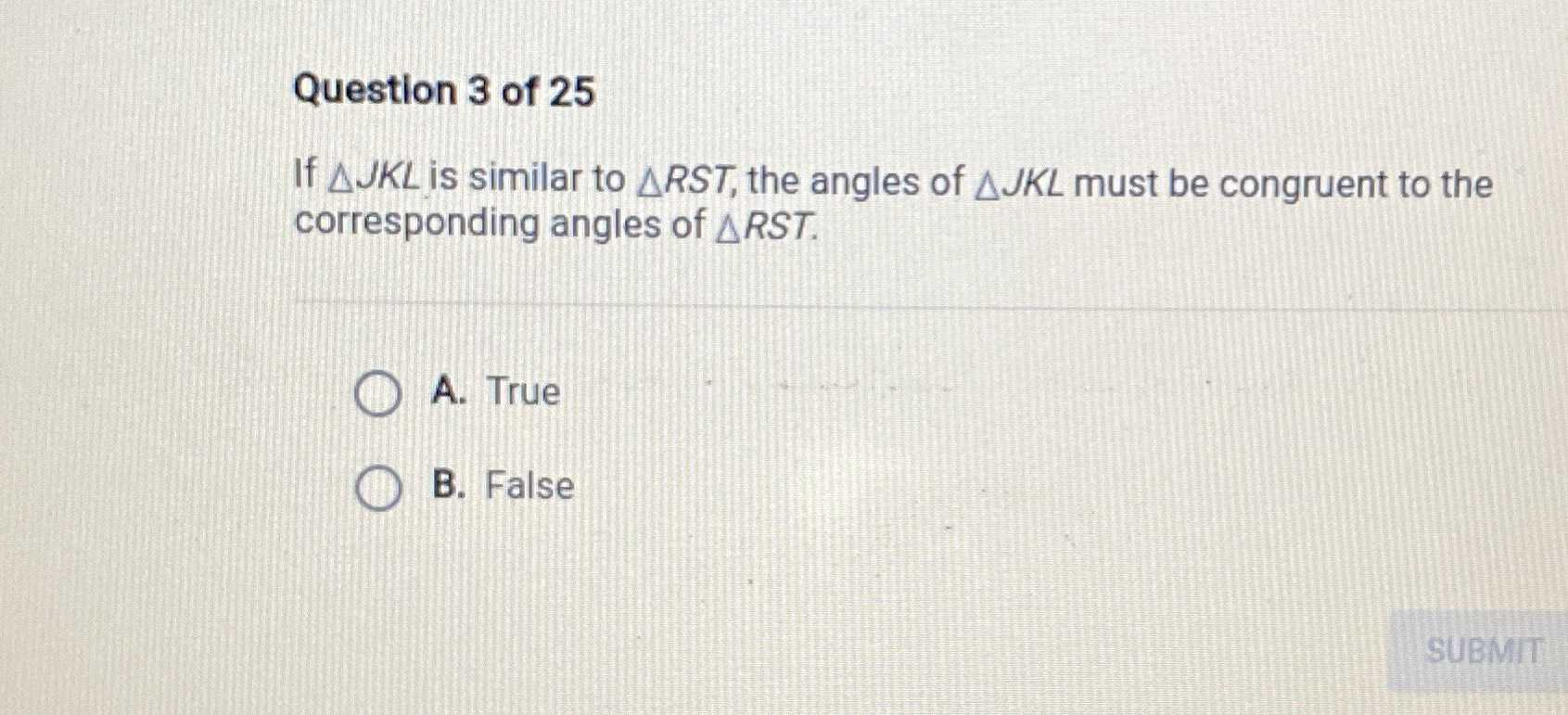### Still have math questions?Question $$3$$ of $$25$$ If $$\triangle J K L$$ is similar to $$\triangle R S T$$ , the angles of $$\triangle J K L$$ must be congruent to the corresponding angles of $$\triangle R S T$$ . True B. False A. TB